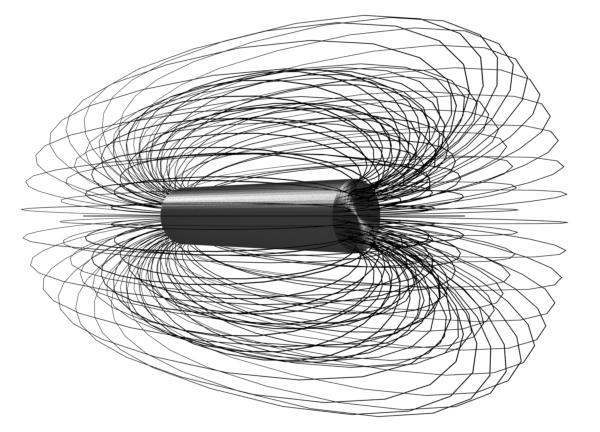# Flux: What is it And The Types of Flux

Contents

## What is Flux?

In physics, Flux is defined as any effect that appears to pass or travel (whether it actually moves or not) through a surface or substance. Flux can be thought of as imaginary lines through which a physical quantity can travel. Flux is a concept in applied mathematics and vector calculus that has many applications to physics.

For transport phenomena, flux is a vector quantity, describing the magnitude and direction of the flow of a property or substance.

In vector calculus flux is a scalar quantity, defined as the surface integral of the perpendicular component of a vector field over a surface.

The word “Flux” is originated from the Latin Word ‘Fluxus’ which means flow. Isaac Newton first used this in differential calculus under the name “fluxion”.

## Types of Flux

The types of flux include:

• Magnetic Flux
• Electric Flux
• Luminous Flux
• Radiant Flux or Energy Flux
• Heat Flux
• Mass Flux
• Momentum Flux
• Acoustic Flux

These types of flux have been explained in more detail.

### Magnetic Flux

Magnetic flux refers to the number of magnetic field lines passing through a closed surface. Its SI unit is – Weber and in CGS is – Maxwell. It is denoted by φM.### Electric Flux

Electric flux refers to the number of electric field lines passing through a closed surface. Its SI unit is – voltmeter. It is denoted as φE.

### Luminous Flux

Luminous flux refers to the line of transmitted visually sensible radiated energy per second. Its SI unit is Lumen (lm).

### Radiant Flux or Energy Flux

Radiant flux refers to the line of transmitted radiated energy per second from any energy source. Its SI unit is Watt. It is denoted as φV.

### Heat Flux

Heat flux refers to the heat energy transfer through an elementary surface per second. Its SI unit is W/m2. It is denoted as Φq.

### Mass Flux

Mass flux refers to the rate of mass flow per unit area. Its SI unit is kg/s-m2. It is denoted as J or q.

### Momentum Flux

Momentum flux refers to the flow of momentum across unit area per second. Its SI unit is N/m2.

### Acoustic Flux

Acoustic refers to the flow of sound energy through the unit area per second. Its SI unit is Watt.

Want To Learn Faster? 🎓
Get electrical articles delivered to your inbox every week.
No credit card required—it’s 100% free.1.